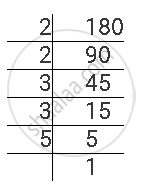Share

# For the Following Number, Find the Smallest Whole Number by Which It Should Be Multiplied So as to Get a Perfect Square Number. Also Find the Square Root of the Square Number So Obtained. 180 - CBSE Class 8 - Mathematics

ConceptSquare Roots - Finding Square Root Through Prime Factorisation

#### Question

For the following number, find the smallest whole number by which it should be multiplied so as to get a perfect square number. Also find the square root of the square number so obtained.

180

#### Solution

180 can be factorised as follows.180 = 2 × 2 × 3 × 3 × 5

Here, prime factor 5 does not have its pair. If 5 gets a pair, then the number will become a perfect square. Therefore, 180 has to be multiplied with 5 to obtain a perfect square.

180 × 5 = 900 = 2 × 2 × 3 × 3 × 5 × 5

Therefore, 180 × 5 = 900 is a perfect square.

∴ sqrt(900) 2 x 3 x 5 = 30

Is there an error in this question or solution?

#### APPEARS IN

NCERT Solution for Mathematics Textbook for Class 8 (2018 to Current)
Chapter 6: Squares and Square Roots
Ex. 6.30 | Q: 5.2 | Page no. 102
Solution For the Following Number, Find the Smallest Whole Number by Which It Should Be Multiplied So as to Get a Perfect Square Number. Also Find the Square Root of the Square Number So Obtained. 180 Concept: Square Roots - Finding Square Root Through Prime Factorisation.
S